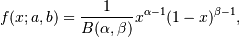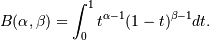# numpy.random.RandomState.beta¶

RandomState.beta(a, b, size=None)

Draw samples from a Beta distribution.

The Beta distribution is a special case of the Dirichlet distribution, and is related to the Gamma distribution. It has the probability distribution functionwhere the normalisation, B, is the beta function,It is often seen in Bayesian inference and order statistics.

Parameters: a : float Alpha, non-negative. b : float Beta, non-negative. size : int or tuple of ints, optional Output shape. If the given shape is, e.g., (m, n, k), then m * n * k samples are drawn. Default is None, in which case a single value is returned. out : ndarray Array of the given shape, containing values drawn from a Beta distribution.

#### Previous topic

numpy.random.RandomState

#### Next topic

numpy.random.RandomState.binomial